# Capacitance - Spherical Conductor, Cylindrical Conductor

CAPACITANCE

Capacitance of a conductor is a measure of ability of the conductor to store charge on it. When a conductor is charged then its potential rises. The increase in potential is directly proportional to the charge given to the conductor.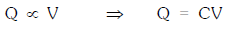The constant C is known as the capacity of the conductor.

Capacitance is a scalar quantity with dimension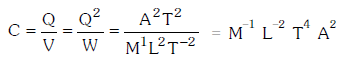The capacity of a conductor is independent of the charge given or its potential raised. It is also independent of nature of material and thickness of the conductor. Theoretically infinite amount of charge can be given to a conductor. But practically the electric field becomes so large that it causes ionisation of medium surrounding it. The charge on conductor leaks reducing its potential.

CAPACITANCE OF A SPHERICAL CONDUCTOR

When a charge Q is given to an isolated spherical conductor then its potential rises.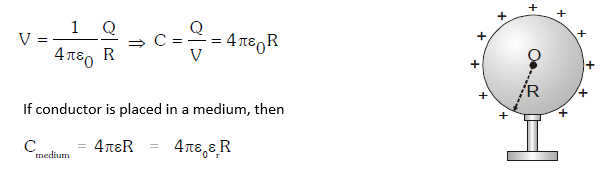Capacitance depends upon:

• Size and Shape of Conductor

• Surrounding medium

• Presence of other conductors nearby

CAPACITANCE OF A CYLINDRICAL CONDUCTOR

When a charge Q is given to inner cylinder it is uniformly distributed on its surface. A charge –Q is induced on inner surface of outer cylinder. The charge +Q induced on outer surface of outer cylinder flows to earth as it is grounded.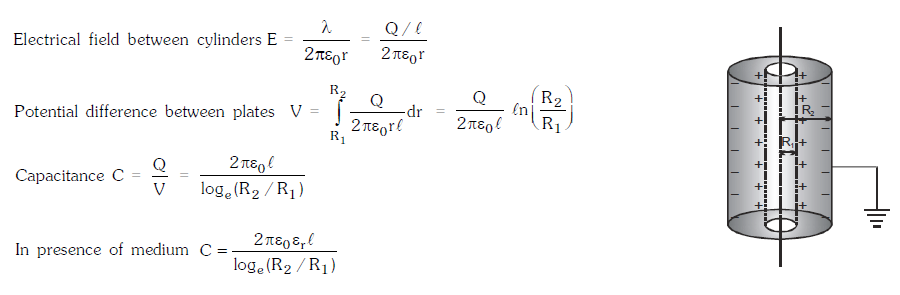CAPACITANCE OF A PAR ALLEL PL ATE CONDUCTOR

It consists of two metallic plates M and N each of area A at separation d. Plate M is positively charged and plate N is earthed. If r is the dielectric constant of the material medium and E is the field at a point P that exists between the two plates, then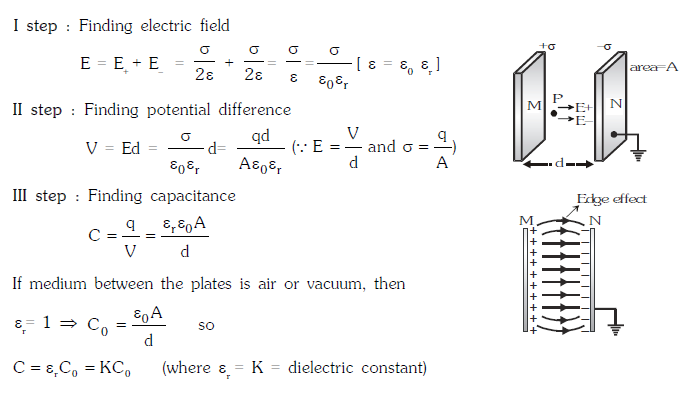Post By : Deepika Singh 25 Dec, 2018 3829 views Physics